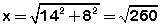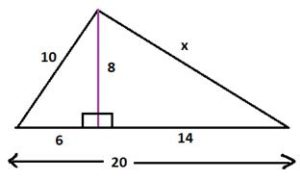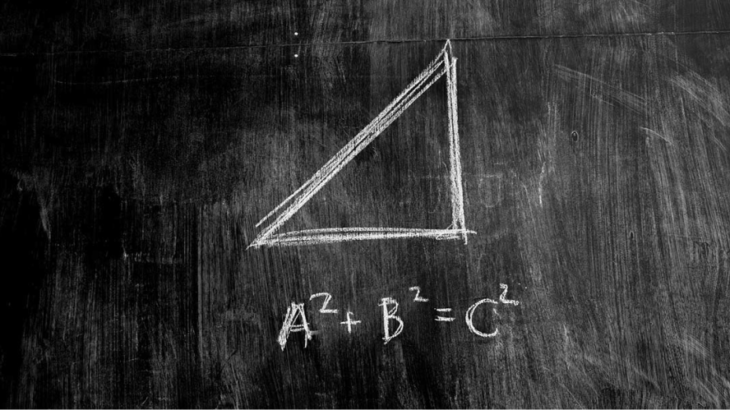# Pythagorean Triplets: Concepts Tricks and CAT Problems

Pythagorean Triplets: Concepts Tricks and CAT Problems
4.7 (94.4%) 25 vote[s]

If we scan the past CAT questions, then it is quite evident that most of the problems related to triangle required Pythagorean triplets as one of the concepts to solve them. It is such an essential theorem because it applies to a wide variety of questions.

In this article will be dealing with the Pythagoras theorem in general and Pythagorean triplets in particular.

Pythagoras Theorem: We all know that if a, b, and c, are the sides of a right triangle, where ‘c’ is the largest side or the hypotenuse of a right triangle, then as per the Pythagoras Theorem we have the following relation: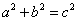Pythagorean Triplets are the set of integers which satisfy the property that they are the sides of a right angle triangle. In other words, the three integers satisfy Pythagoras theorem.

Triangle with side 3, 4 and 5 is the smallest right triangle which has all three sides and integer. So, we can say that (3, 4, 5) is one of the triplets. There exist infinite such triplets. Below is the list of triplets which commonly appear in competitive exams like CAT.

## List of Pythagorean Triplets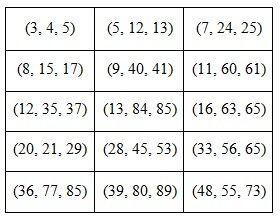Important: If a, b, c is a Pythagorean triplet, then ka, kb, kc will also form a Pythagorean triplet; where k is any positive integer. For example, (3, 4, 5) is a triplet, then (6,8,10), (9,12,15),(12,16,20) etc. will also be triplets.

It is advisable that a student must learn these triplets to use them effectively.  At least the first five should be at your fingertips. However, there is a method to generate triplet if we know the smallest side of the triplet. Let us understand the method of generating these triplets

## Methods to generate Pythagorean Triplets

There are two different methods of generating triplets based on whether the smallest side is odd or even.

### If the smallest side is ODD

We follow the following steps to generate the other two sides of the integer if the smallest  side (odd) is known:

Step 1: Take the square of the sides. And let this square be x.

Step 2:  Split the number x into two parts such that the difference between them is 1, i.e.,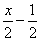and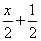. These two parts will be the other two sides of the triplets.

Let’s take an example to understand the steps:

Let us generate the triplets 3, 4, and 5 using these steps.

Step 1:  Squaring 3, we get 9.

Step 2:  Splitting 9 into two parts such that their difference between them is 1, so, the parts would be 4 and 5 respectively.

Here we can see that the other two sides are 4 and 5.

### If the smallest side is EVEN

The following steps are followed to generate triplets if the smallest side is even. Let the smallest side be x.

Step 1: Calculate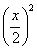Step 2: the two sides would be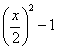, and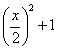Let us also understand the application with the help of an example.

We would generate the other two sides of a right angle triangle whose smallest size is 8.

Step 1: calculating (8/2)2=16

Step 2:  the required sides  would be (16-1=15) and (16+1=17)

Important: (20, 21, 29) is also a Pythagorean Triplet, though it cannot be generated using the above method.

We can observe that these methods are straightforward and required only two steps.

## Pythagorean Triplets based CAT problems

Question: A ladder leans against a vertical wall. The top of the ladder is 8 m above the ground. When the bottom of the ladder is moved 2 m farther away from the wall, the top of the ladder rests against the foot of the wall. What is the length of the ladder? [ CAT 2001 ]

Explanation: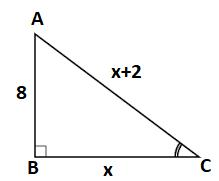The question can be visualized as shown above. On dragging the foot of the latter (point C) 2 m away from point B, point A coincides with point B.

Hence, we can say that AC = BC + 2.

Further, if we observe closely, then we find that the Pythagorean triplet with one of the side 8 is (8, 15, 17). Here, if we consider AB=8, BC =15, and AC=17, then the values perfectly agrees with the condition. Therefore, the length of the ladder is 17 m.

Question: The length of a ladder is precisely equal to the height of the wall it is resting against. If the lower end of the ladder is kept on a stool of length 3m and the stool is kept 9 m away from the wall, and the upper end of the ladder coincides with the tip of the wall. Then, the height of the wall is: [ CAT 1995 ]

Explanation: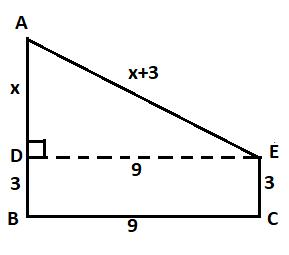Refer to the figure above. From the conditions given in the question, it is clear that AB (wall) = AE (ladder) where BC=DE. So, we have right triangle ADE with sides x, 9, and x+3.

From here we have two options, one is to apply Pythagoras theorem and get the value of x, the other is to figure out triplet which fits the condition.

Here one of the sides is 9. So, the possible triplets could be (9, 40, 41) and (9, 12, 15). Clearly, (9, 40, 41) can be discarded because if we take x =40 then x+3 ≠ 41.

Hence, the only possibility is (9, 12, 15). Here we can see that if we consider x =12, then x+3 = 15 fits with the condition.

Therefore, the answer to this question is 15 m.

Question: Euclid has a triangle in mind. Its longest side has length 20, and another of its sides has length 10. Its area is 80. What is the exact length of its third side? [ CAT 2001 ]

Explanation:

From the given conditions, we can draw a rough diagram of the triangle as provided below: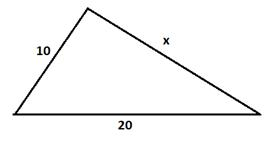It is interesting to observe here is that the area of the triangle is 80 and if we consider side with length 20 as the base of the triangle, then we will get the height too as an integer, i.e.,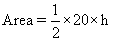Refer to the diagram below, its clear that if we apply Pythagoras theorem, we get